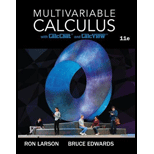# Converting Coordinates In Exercises 41-44, convert the integral from rectangular coordinates to both cylindrical and spherical coordintates, and evaluate the simplest iterated integral. ∫ 0 2 ∫ 0 4 − x 2 ∫ 0 16 − x 2 − y 2 x 2 + y 2 d z d y d x### Multivariable Calculus

11th Edition
Ron Larson + 1 other
Publisher: Cengage Learning
ISBN: 9781337275378

#### Solutions

Chapter
Section### Multivariable Calculus

11th Edition
Ron Larson + 1 other
Publisher: Cengage Learning
ISBN: 9781337275378
Chapter 14.7, Problem 42E
Textbook Problem
1 views

## Converting Coordinates In Exercises 41-44, convert the integral from rectangular coordinates to both cylindrical and spherical coordintates, and evaluate the simplest iterated integral. ∫ 0 2 ∫ 0 4 − x 2 ∫ 0 16 − x 2 − y 2 x 2 + y 2 d z d y d x

This textbook solution is under construction.

### Still sussing out bartleby?

Check out a sample textbook solution.

See a sample solution

#### The Solution to Your Study Problems

Bartleby provides explanations to thousands of textbook problems written by our experts, many with advanced degrees!

Get Started